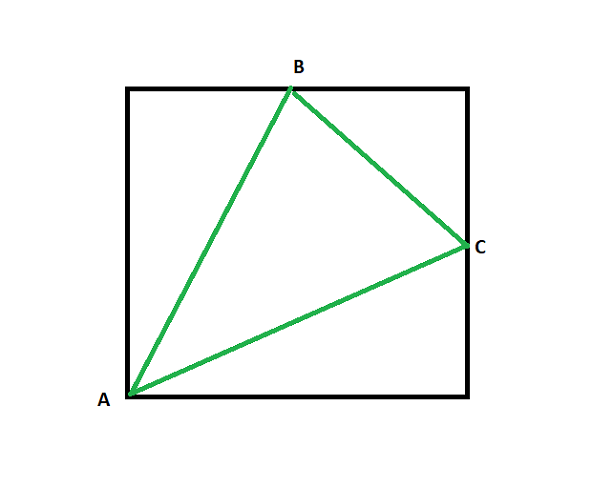# Area of a triangle with two vertices at midpoints of opposite sides of a square and the other vertex lying on vertex of a square

• Last Updated : 11 Jun, 2021

Given a positive integer N representing the side of a square, the task is to find the area of a triangle formed by connecting the midpoints of two adjacent sides and vertex opposite to the two sides.

Examples:

Attention reader! Don’t stop learning now. Get hold of all the important DSA concepts with the DSA Self Paced Course at a student-friendly price and become industry ready.  To complete your preparation from learning a language to DS Algo and many more,  please refer Complete Interview Preparation Course.

In case you wish to attend live classes with experts, please refer DSA Live Classes for Working Professionals and Competitive Programming Live for Students.

Input: N = 10
Output: 37.5

Input: N = 1
Output: 0.375

Approach: The given problem can be solved based on the following observations:• The one side of the triangle will be the hypotenuse of the triangle formed with the vertices as two middle point and one vertex of the square at the intersection of the sides whose length of the side is given by.
• The length of the other two sides of the triangle is given by.
• Now, the sides of the triangle are known, therefore, the area of the triangle can be calculated using the Heron’s Formula.

Follow the steps below to solve the problem:

Below is the implementation of the above approach:

## C++

 // C++ program for the above approach #include using namespace std; // Function to find the area of the// triangle that inscribed in squaredouble areaOftriangle(int side){    // Stores the length of the first    // side of triangle    double a = sqrt(pow(side / 2, 2)                    + pow(side / 2, 2));     // Stores the length of the second    // side of triangle    double b = sqrt(pow(side, 2)                    + pow(side / 2, 2));     // Stores the length of the third    // side of triangle    double c = sqrt(pow(side, 2)                    + pow(side / 2, 2));     double s = (a + b + c) / 2;     // Stores the area of the triangle    double area = sqrt(s * (s - a)                       * (s - b) * (s - c));     // Return the resultant area    return area;} // Driver Codeint main(){    int N = 10;    cout << areaOftriangle(N);     return 0;}

## Java

 // Java program for the above approachimport java.util.*; class GFG{  // Function to find the area of the// triangle that inscribed in squarestatic double areaOftriangle(int side){         // Stores the length of the first    // side of triangle    double a = Math.sqrt(Math.pow(side / 2, 2) +                         Math.pow(side / 2, 2));     // Stores the length of the second    // side of triangle    double b = Math.sqrt(Math.pow(side, 2) +                         Math.pow(side / 2, 2));     // Stores the length of the third    // side of triangle    double c = Math.sqrt(Math.pow(side, 2) +                         Math.pow(side / 2, 2));     double s = (a + b + c) / 2;     // Stores the area of the triangle    double area = Math.sqrt(s * (s - a) *                           (s - b) * (s - c));     // Return the resultant area    return area;}  // Driver codepublic static void main(String[] args){    int N = 10;         System.out.print(areaOftriangle(N));}} // This code is contributed by sanjoy_62

## Python3

 # Python3 program for the above approachfrom math import sqrt # Function to find the area of the# triangle that inscribed in squaredef areaOftriangle(side):         # Stores the length of the first    # side of triangle    a = sqrt(pow(side / 2, 2) + pow(side / 2, 2))     # Stores the length of the second    # side of triangle    b = sqrt(pow(side, 2) + pow(side / 2, 2))     # Stores the length of the third    # side of triangle    c = sqrt(pow(side, 2) + pow(side / 2, 2))     s = (a + b + c) / 2     # Stores the area of the triangle    area = sqrt(s * (s - a) * (s - b) * (s - c))     # Return the resultant area    return round(area, 1) # Driver Codeif __name__ == '__main__':         N = 10         print (areaOftriangle(N)) # This code is contributed by mohit kumar 29

## C#

 // C# program for the above approachusing System;class GFG{  // Function to find the area of the// triangle that inscribed in squarestatic double areaOftriangle(int side){         // Stores the length of the first    // side of triangle    double a = Math.Sqrt(Math.Pow(side / 2, 2) +                         Math.Pow(side / 2, 2));     // Stores the length of the second    // side of triangle    double b = Math.Sqrt(Math.Pow(side, 2) +                         Math.Pow(side / 2, 2));     // Stores the length of the third    // side of triangle    double c = Math.Sqrt(Math.Pow(side, 2) +                         Math.Pow(side / 2, 2));     double s = (a + b + c) / 2;     // Stores the area of the triangle    double area = Math.Sqrt(s * (s - a) *                           (s - b) * (s - c));     // Return the resultant area    return area;}  // Driver codepublic static void Main(string[] args){    int N = 10;         Console.WriteLine(areaOftriangle(N));}} // This code is contributed by ukasp.

## Javascript

 
Output:
37.5

Time Complexity: O(1)
Auxiliary Space: O(1)

My Personal Notes arrow_drop_up